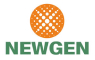New update is available. Click here to update.

# Sort 0s, 1s, 2s

Contributed by
Utkarsh Kumar Choubey
Easy0/402 upvotes+3 more

## Problem Statement

#### For Example:

``````For ‘arr’ = {1, 0, 0, 2, 1}.
‘Answer’ = {0, 0, 1, 1, 2}.
‘Answer’ should contain all 0s first, then all 1s and all 2s in the end.
``````
Detailed explanation ( Input/output format, Notes, Images )
##### Constraints:
``````1 <=  ‘T’ <= 10^5
1 <= ‘N’ <= 10^5
0 <= ‘arr[i]’ <= 2
Sum of ‘N’ for all testcases <= 10^5

Time Limit: 1 sec
``````
##### Sample Input 1:
``````2
4
1 2 0 1
3
1 0 1
``````
##### Sample Output 1:
``````0 1 1 2
0 1 1
``````
##### Explanation of sample output 1:
``````For Test 1:
For ‘arr’ = {1, 2, 0, 1}.
‘Answer’ = {0, 1, 1, 2}.
In ‘Answer all 0 is placed first, then all the 1s and finally 2.

For Test 2:
For ‘arr’ = {1, 0, 1}.
In ‘Answer all 0 is placed first, then all the 1s.
``````
##### Sample Input 2:
``````2
4
0 1 1 2
3
2 1 1
``````
##### Sample Output 2:
``````0 1 1 2
1 1 2
``````AutocompleteConsole